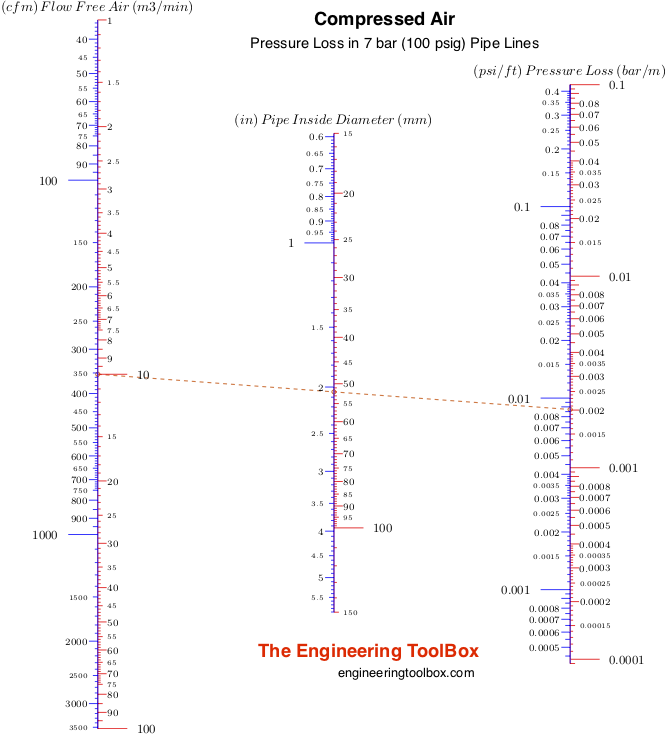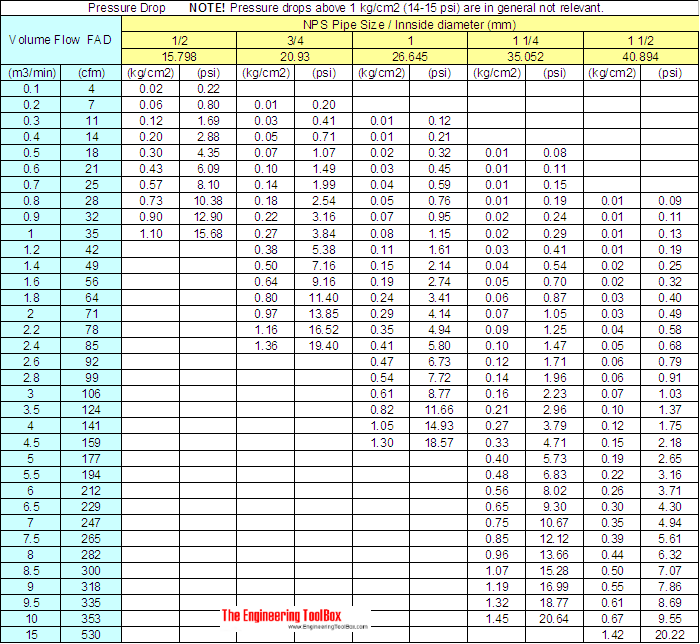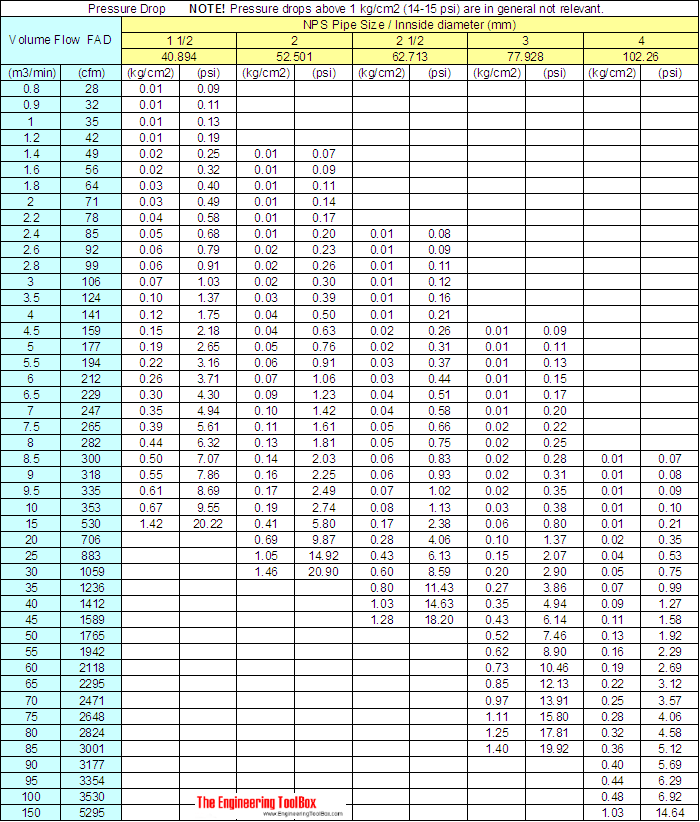Engineering ToolBox - Resources, Tools and Basic Information for Engineering and Design of Technical Applications!

# Compressed Air - Pipe Line Pressure Loss - Online Calculator with Metric and Imperial Units

## Calculate pressure drop in compressed air pipe lines - metric and imperial units.

The pressure drop in compressed air lines can be calculated with the empirical formula

dp = 7.57 q1.85 L 104 / (d5 p)                                             (1)

where

dp = pressure drop (kg/cm2)

q = air volume flow at atmospheric conditions (FAD) (m3/min)

L = length of pipe (m)

d = inside diameter of pipe (mm)

p = initial pressure - gauge (kg/cm2)

• 1 kg/cm2 = 98068 Pa = 0.98 bar = 0.97 atmosphere = 736 mm Hg = 10000 mm H2O = 10 m H2O = 2050 psf = 14.2 psi = 29 in Hg = 394 in H2O = 32.8 ft H2O

Note! - pressure is "force per unit area" and commonly used pressure units like kg/cm2 and similar are in principle not correct since kg is a mass unit. A mass must be multiplied with gravity g to be a force (weight).

### Compressed Air - Pressure Drop Nomogram

The nomogram below can be used to estimate pressure drops in compressed air pipe lines with pressure 7 bar (100 psig).### Online Compressed Air Pipeline Pressure Drop Calculator - Metric Units

The calculator below can used to calculate the pressure drop in compressed air pipelines.

q - air volume flow at atmospheric conditions (FAD) (m3/min)

L - pipe length (m)

p - initial gauge pressure (kg/cm2)

### Online Compressed Air Pipeline Pressure Drop Calculator - Imperial Units

The calculator below can used to calculate the pressure drop in compressed air pipelines.

q - air volume flow at atmospheric conditions (FAD) (cfm)

L - pipe length (feet)

p - initial gauge pressure (psig)

NOTE! - a pressure drop above 1 kg/cm2 (14-15 psi) is in general not relevant and the formula and calculators above may not be valid.

For a more accurate calculation - or for a longer pipe lines with larger pressure drops - divide the line in parts and calculate the pressure drop and final pressure for each part. Use final pressures as initial pressures for the next parts. The final pressure after the last part is the final pressure at the end of the pipe line. The pressure drop for the whole pipe line can also be calculated by summarizing the pressure drops for each part.

### Compressed Air Pipeline Pressure Drop Spreadsheet

Calculations can be done for other pressures and/or pipe lengths by using this excel spreadsheet (metric units).

Or, alternatively - Compressed air pipe lines - pressure drop calculations - in Google Docs. You can open, save and modify your own copy of the Google spreadsheet if you are signed into your Google Account.

### Compressed Air Pipeline Pressure Drop Table - Initial gauge Pressure 7 kg/cm2 (100 psig)

Pressure drops in 100 m (330 ft) compressed air schedule 40 steel pipe lines are indicated in the tables below:## Engineering ToolBox - SketchUp Extension - Online 3D modeling!

Add standard and customized parametric components - like flange beams, lumbers, piping, stairs and more - to your Sketchup model with the Engineering ToolBox - SketchUp Extension - enabled for use with the amazing, fun and free SketchUp Make and SketchUp Pro .Add the Engineering ToolBox extension to your SketchUp from the SketchUp Pro Sketchup Extension Warehouse!

Translate

## Privacy

We don't collect information from our users. Only emails and answers are saved in our archive. Cookies are only used in the browser to improve user experience.

Some of our calculators and applications let you save application data to your local computer. These applications will - due to browser restrictions - send data between your browser and our server. We don't save this data.

## Citation

• Engineering ToolBox, (2005). Compressed Air - Pipe Line Pressure Loss - Online Calculator with Metric and Imperial Units. [online] Available at: https://www.engineeringtoolbox.com/pressure-drop-compressed-air-pipes-d_852.html [Accessed Day Mo. Year].

Modify access date.

. .

#### Scientific Online Calculator6 24